# Gauthmath-New Smart CalculatorAre you looking for a math homework answer scanner?
Need help to solve math problems with real math tutors?

Download Gauthmath – the powerful math problem solver with no cost! Our mathematics app gives access to online real math tutors for algebra, graphing, calculus, even math word problems, and all other math problems!
Simply snap a photo of your question with our math homework solver app and get step-by-step answers instantly.

◉【WORD PROBLEM FULLY COVERED】Gauthmath is your math word problem solver that can solve even the hardest math word problems. The real math tutors online on our mathematics solution app, give answers with step-by-step explanations to all your maths questions and math homework.

◉【AMAZING MATH PROBLEM SOLVER】As one of the powerful math apps that give you the answer, Gauthmath can help and solve all your math homework. It's like you have a photo math question solver camera. Just take a photo or scan your maths homework, the Gauthmath math scanner & math answer app will instantly get you the math problem answers to any math questions!

◉【MATH ANSWER IN SECONDS】Besides allowing you to easily scan math problems, Gauthmath maths homework solver app has thousands of helpers and math solution experts. Once they got your math problem photo, the dedicated math helper will answer instantly with a step-by-step solution. Get math answers anywhere, anytime, 24/7.

◉【EASY-TO-USE MATH SCANNER】Gauthmath math problem solver app can help you solve all kinds of math problems! Even the hardest types of problems. It covers all kinds of math problems IB/ A Level/ SAT / ACT / AP / GCSE / HSC math and other advanced mathematics.

►MAIN FEATURES
· Solve math problems instantly with a math tutoring expert!
· LIVE Math experts available 24/7!
· Math solutions with step-by-step explanations!
· Gauthmath solves word problems even the hard ones!
· Snap a picture math question, and you will get the problem solved in seconds!

►MATH TOPICS COVERED
· Math Word problems
· Algebra (Real Number; Arithmetic; Set theory; Expression; Logarithm; Complex Number)
· Function (Linear; Quadratic; Polynomial; Exponential; Rational; Logarithmic; Inverse Function)
· Geometry (Plane & Solid Geometry; Algebra & lines; lines and planes in space; transformation)
· Trigonometry (Trigonometric Ratios; Law of Sines; Law of Cosines; Reciprocal Properties)
· Calculus (Derivatives; Integrals; Limits; the tangent/area problem)
· Statistics and Data Analysis (Probability; data representation; random variables; binomial theorem; Poisson distribution; normal distributions; confidence intervals)
· Matrix (matrix algebra; the system of linear equations and matrices)
· Logic (reasoning and proof; conditional statements; inductive reasoning; deductive reasoning)

Membership auto-renewal subscription instructions
Monthly: 1 month, \$4.99 first month then \$6.99
Quarterly: 3 months, \$15.99
Annual: 12 months, \$49.99
– Payment: Continuous monthly membership is an auto-renewing item, users will be credited to their iTunes account after confirming purchase and payment.
– Renewal: Apple iTunes account will be charged within 24 hours before the expiration date, and the subscription period will be extended by one subscription period after the successful charge.
– To cancel the subscription: Open "Settings" on your Apple phone → Tap your name → Tap Subscriptions → Tap the subscription that you want to manage → Tap Cancel Subscription.
—————–

◆【STAY CONNECTED】
►Tiktok: https://www.tiktok.com/@gauthmath_official
►Discord: https://discord.gg/cy6jtuY9At
►Website: www.gauthmath.com
►Email: [email protected]

Price:Free## Evaluation at the itunes store

Evaluation of iPhone App [Gauthmath-New Smart Calculator] at itunes store

• newest version:(4 / 5)4.02604
• All versions:(4 / 5)4.0

Number of people evaluated:3955

Price:Free

### ScreenShots

Screenshots of iPhone Apps [Gauthmath-New Smart Calculator]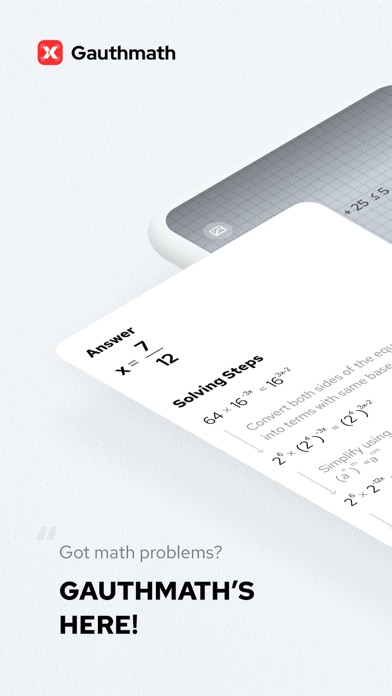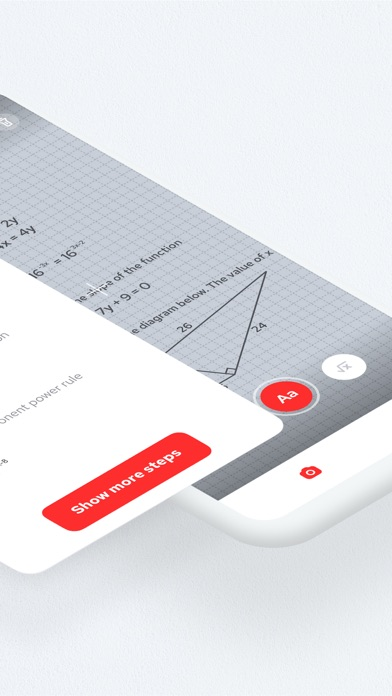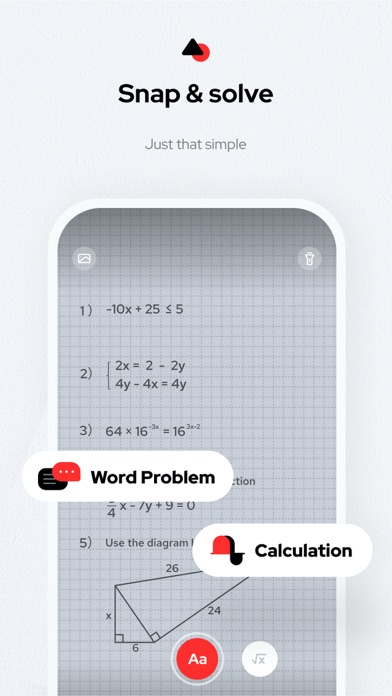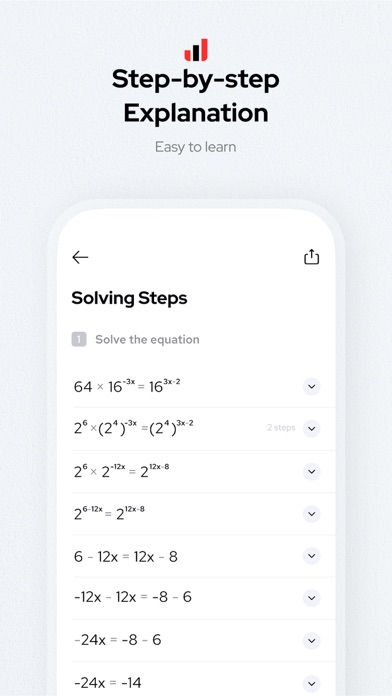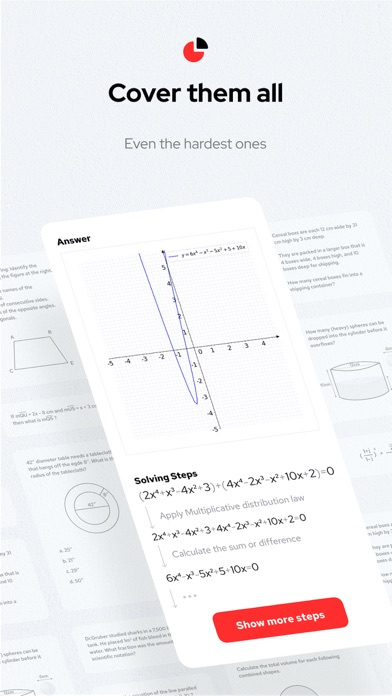(c)GAUTHTECH PTE. LTD.

## People reviews

Impression and review of people to iPhone App [Gauthmath-New Smart Calculator]!

### Change log

Update history of iPhone App [Gauthmath-New Smart Calculator]

Ahooy! We are here with a fantastic new feature: Question Bank!
You can now keep track of all the questions with wrong answers or misunderstood concepts and then refer back to them as you study; categorize the questions based on your level and what mistakes you made; practice with similar questions to strengthen your math skills!
With Question Bank, practice and review is just cool and easy! Let’s smash some real math problems together! Cheers!
(c)GAUTHTECH PTE. LTD.

### details

iPhone App [Gauthmath-New Smart Calculator] Other details

• Sales maker:
GAUTHTECH PTE. LTD.
• Release date:
• Version:1.9.1
• Price:Free